×#### Thank you for registering.

One of our academic counsellors will contact you within 1 working day.

Click to Chat

1800-1023-196

+91-120-4616500

CART 0

• 0

MY CART (5)

Use Coupon: CART20 and get 20% off on all online Study Material

ITEM
DETAILS
MRP
DISCOUNT
FINAL PRICE
Total Price: Rs.

There are no items in this cart.
Continue Shopping• Complete JEE Main/Advanced Course and Test Series
• OFFERED PRICE: Rs. 15,900
• View Details

```Congruence Exercise 16.4

Question: 1

Which of the following pairs of triangle are congruent by ASA condition?

Solution:

i)We have,

Since ∠ABO = ∠CDO = 45° and both are alternate angles, AB // DC, ∠BAO = ∠DCO (alternate angle, AB // CD and AC is a transversal line)

∠ABO = ∠CDO = 45° (given in the figure) Also, AB = DC (Given in the figure)

Therefore, by ASA ΔAOB ≅ ΔDOC

ii)In ABC,

Now AB =AC (Given)

∠ABD = ∠ACD = 40° (Angles opposite to equal sides)

∠ABD + ∠ACD + ∠BAC = 180° (Angle sum property)

40° + 40° + ∠BAC=180°

∠BAC =180°- 80° =100°

∠BAD + ∠DAC = ∠BAC ∠BAD = ∠BAC – ∠DAC = 100° – 50° = 50°

Therefore, by ASA, ΔABD ≅ ΔADC

iii)In Δ ABC,

∠A + ∠B + ∠C = 180°(Angle sum property)

∠C = 180°- ∠A – ∠B ∠C = 180° – 30° – 90° = 60°

In PQR,

∠P + ∠Q + ∠R = 180°(Angle sum property)

∠P = 180° – ∠Q – ∠R ∠P = 180°- 60°- 90° = 30°

∠BAC = ∠QPR = 30°

∠BCA = ∠PRQ = 60° and AC = PR (Given)

Therefore, by ASA, ΔABC ≅ ΔPQR

iv)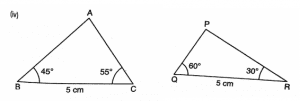We have only

BC = QR but none of the angles of ΔABC and ΔPQR are equal.

Therefore, ΔABC and Cong ΔPRQ

Question: 2

(ii) State the three pairs of matching parts you have used in (i)

(iii) Is it true to say that BD = DC?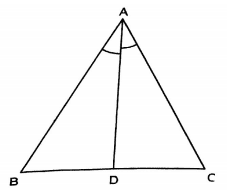Solution:

Question: 3

Draw any triangle ABC. Use ASA condition to construct other triangle congruent to it.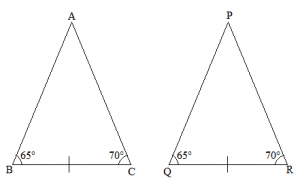Solution:

We have drawn

Δ ABC with ∠ABC = 65° and ∠ACB = 70°

We now construct ΔPQR ≅ ΔABC has ∠PQR = 65° and ∠PRQ = 70°

Also we construct ΔPQR such that BC = QR

Therefore by ASA the two triangles are congruent

Question: 4

In Δ ABC, it is known that ∠B = C. Imagine you have another copy of Δ ABC

(i) Is ΔABC ≅ ΔACB

(ii) State the three pairs of matching parts you have used to answer (i).

(iii) Is it true to say that AB = AC?Solution:

(i) Yes ΔABC ≅ ΔACB

(ii) We have used ∠ABC = ∠ACB and ∠ACB = ∠ABC again.

Also BC = CB

(iii) Yes it is true to say that AB = AC since ∠ABC = ∠ACB.

Question: 5

In Figure, AX bisects ∠BAC as well as ∠BDC. State the three facts needed to ensure that ΔACD ≅ ΔABD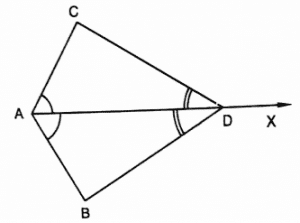Solution:

As per the given conditions, ∠CAD = ∠BAD and ∠CDA = ∠BDA (because AX bisects ∠BAC)

Therefore, by ASA, ΔACD ≅ ΔABD

Question: 6

In Figure, AO = OB and ∠A = ∠B.

(i) Is ΔAOC ≅ ΔBOD

(ii) State the matching pair you have used, which is not given in the question.

(iii) Is it true to say that ∠ACO = ∠BDO?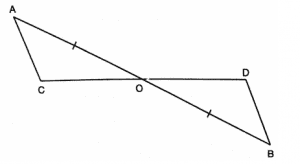Solution:

We have

∠OAC = ∠OBD,

AO = OB

Also, ∠AOC = ∠BOD (Opposite angles on same vertex)

Therefore, by ASA ΔAOC ≅ ΔBOD
```### Course Features

• 728 Video Lectures
• Revision Notes
• Previous Year Papers
• Mind Map
• Study Planner
• NCERT Solutions
• Discussion Forum
• Test paper with Video Solution